Piriform Curve
PIRIFORM CURVE

Balmoral Software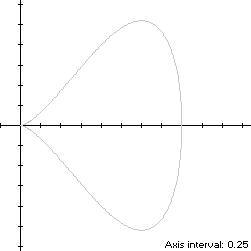The piriform curve is a knob-shaped x-symmetric closed curve with parametric equations
x(t) = 1 + sin(t)

y(t) = [1 + sin(t)]cos(t), 0 ≤ t < 2π

The curve is traced out clockwise as t increases, starting from the point (x(0),y(0)) = (1,1). Its extreme abscissas occur at (2,0) when t = π/2 and at the origin when t = 3π/2. The maximum ordinate of the piriform curve occurs at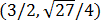when t = π/6, so the width x height of its bounding rectangle is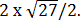### Metrics

We have
x'(t) = cos(t)

y'(t) = cos(2t) - sin(t),

so by (L1), the perimeter of the piriform curve is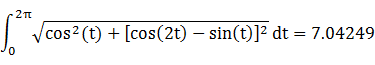and its area is π. By (C1), its centroid abscissa is(Note: WolframAlpha has omitted the division by π.)

### Convex Hull

We have
x''(t) = -sin(t)

y''(t) = -2sin(2t) - cos(t),

so by (X1), the piriform curvature changes sign at the origin since
cos(t)[-2sin(2t) - cos(t)] = -sin(t)[cos(2t) - sin(t)]
is satisfied for t = 3π/2. Equation (X2) is evaluated with respect to the origin as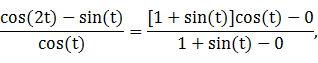which has a solution in the upper half-plane at t = 0, so the convex hull line segments extend between the origin and the points (1,±1). These line segments are shown in blue in the left diagram below, and each has length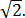By (L1), the perimeter of the convex hull is: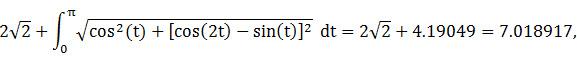which is less than 1% shorter than that of the piriform curve.

The line segments of the convex hull create an isosceles triangle with area 1. The centroid abscissa of this triangle is the average of its vertex abscissas, or 2/3. By (A1), the remainder of the convex hull has area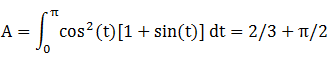By (C1), the associated centroid abscissa is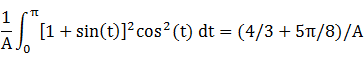The convex hull component metrics can be summarized as follows:
RegionAreaCentroid abscissaProduct
Triangle12/32/3
RemainderA = 2/3 + π/2(4/3 + 5π/8)/A4/3 + 5π/8
Total5/3 + π/22 + 5π/8
The area of the convex hull is 3.237463, about 3% larger than that of the piriform curve. The centroid abscissa of the convex hull is the weighted average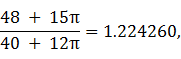which is 1/(20 + 6π) to the left of the centroid of the piriform curve itself, exactly the same distance as the corresponding value for the teardrop curve.

### Incircle

The maximum ordinate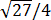of the piriform curve does not define its inradius since the corresponding abscissa 3/2 is too close to the right edge (2,0), so its incircle is constrained by the right edge. Using z = 2 in Lemma C, we have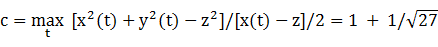and R = |c - z| =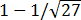. For verification, we have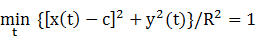### Inellipse

Using z = 2 in Lemma E,
d/dt [x(t) - z]y(t) = d/dt -cos3(t)
has a zero at t* = 0. The corresponding coordinates are
x* = y* = 1,
from which we have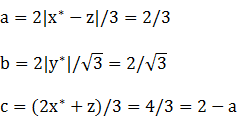For verification, we have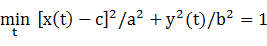### Circumellipse

Using z = 0 in Lemma E,
d/dt [x(t) - z]y(t) = d/dt [1 + sin(t)]2cos(t)
has a zero at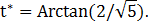The corresponding coordinates are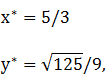from which we have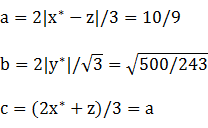For verification, we have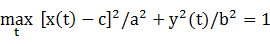### Circumcircle

Using the origin as the reference point in Lemma C, we have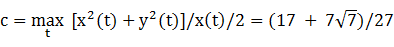and R = |c - z| = c. For verification, we have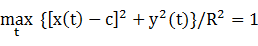### Summary Table

 Perimeter Area Centroid Figure Parameters Incircle R =5.073986 2.048748 (1.192450,0) Inellipse5.825171 2.418399 (1.333333,0) Piriform curve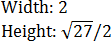7.04249 3.141593 (1.25,0) Convex hull 7.018917 3.237463 (1.224260,0) Circumellipse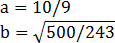8.029365 5.007134 (1.111111,0) Circumcircle R =8.265940 5.437191 (1.315565,0)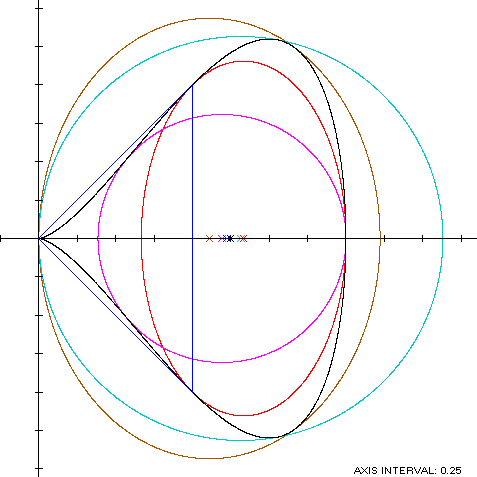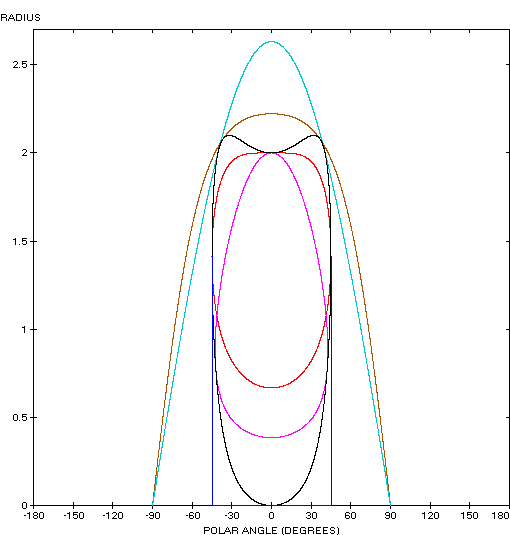The piriform curve (red) is a member of a group of similarly-shaped figures described on these pages, including (inside to outside) the teardrop curve, the Tschirnhausen cubic, the right strophoid and the Trisectrix of Maclaurin: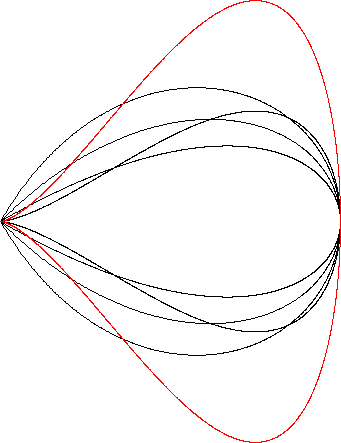The piriform curve adjoins and is the same height as the cardioid:### Relationship with the teardrop curve

See the section on the teardrop curve page.